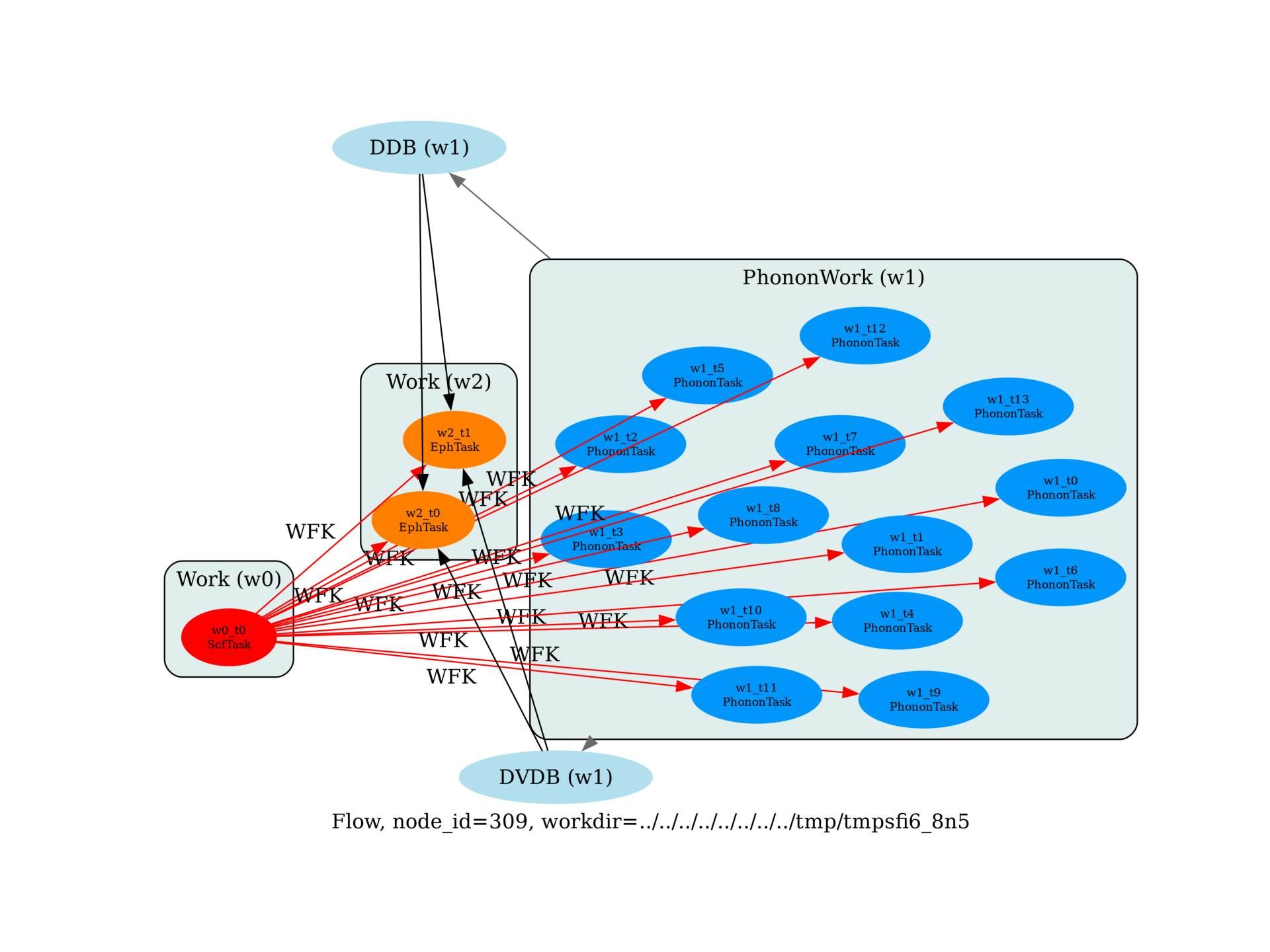Flow for E-PH calculations¶

This flow computes the phonon linewidths and the Eliashberg function in Al.import sys
import os
import abipy.data as abidata
import abipy.abilab as abilab
import abipy.flowtk as flowtk

def build_flow(options):
# Working directory (default is the name of the script with '.py' removed and "run_" replaced by "flow_")
if not options.workdir:
options.workdir = os.path.basename(sys.argv).replace(".py", "").replace("run_", "flow_")

# Preparatory run for E-PH calculations.
# The sequence of datasets makes the ground states and
# all of the independent perturbations of the single Al atom
# for the irreducible qpoints in a 4x4x4 grid.
# Note that the q-point grid must be a sub-grid of the k-point grid (here 8x8x8)
pseudos = abidata.pseudos("Al.oncvpsp")

structure = abilab.Structure.from_abivars(
acell=3 * [7.5],
rprim=[0.0, 0.5, 0.5,
0.5, 0.0, 0.5,
0.5, 0.5, 0.0],
typat=1,
xred=[0.0, 0.0, 0.0],
ntypat=1,
znucl=13,
)

same_structure = abilab.Structure.fcc(a=7.5, species=["Al"], units="bohr")
assert same_structure == structure

gs_inp = abilab.AbinitInput(structure, pseudos)

gs_inp.set_vars(
ecut=8.0,
nband=5,
occopt=7,    # include metallic occupation function with a small smearing
tsmear=0.04,
tolvrs=1e-7,
)

# The kpoint grid is minimalistic to keep the calculation manageable.
gs_inp.set_kmesh(
ngkpt=[8, 8, 8],
shiftk=[0.0, 0.0, 0.0],
)

# Phonon calculation with 4x4x4
ddb_ngqpt = [4, 4, 4]
qpoints = gs_inp.abiget_ibz(ngkpt=ddb_ngqpt, shiftk=[0, 0, 0], kptopt=1).points

flow = flowtk.Flow(options.workdir, manager=options.manager)

flow.register_work(ph_work)

eph_work = flow.register_work(flowtk.Work())
eph_deps = {work0: "WFK", ph_work: ["DDB", "DVDB"]}

for eph_ngqpt_fine in [(4, 4, 4,), (8, 8, 8)]:
# Build input file for E-PH run.
eph_inp = gs_inp.new_with_vars(
optdriver=7,
ddb_ngqpt=ddb_ngqpt,           # q-mesh used to produce the DDB file (must be consistent with DDB data)
eph_intmeth=2,                 # Tetra method
eph_fsewin="0.8 eV",           # Energy window around Ef
eph_mustar=0.12,               # mustar parameter
eph_ngqpt_fine=eph_ngqpt_fine, # Interpolate DFPT potentials if != ddb_ngqpt
)

# Set q-path for phonons and phonon linewidths.
eph_inp.set_qpath(20)

# TODO: Define wstep and smear
# Set q-mesh for phonons DOS and a2F(w)
eph_inp.set_phdos_qmesh(nqsmall=16, method="tetra")

flow.allocate(use_smartio=True)

return flow

# This block generates the thumbnails in the AbiPy gallery.
# You can safely REMOVE this part if you are using this script for production runs.
__name__ = None
import tempfile
options = flowtk.build_flow_main_parser().parse_args(["-w", tempfile.mkdtemp()])
build_flow(options).graphviz_imshow()

@flowtk.flow_main
def main(options):
"""
This is our main function that will be invoked by the script.
flow_main is a decorator implementing the command line interface.
Command line args are stored in `options`.
"""
return build_flow(options)

if __name__ == "__main__":
sys.exit(main())

Total running time of the script: ( 0 minutes 1.650 seconds)

Gallery generated by Sphinx-Gallery